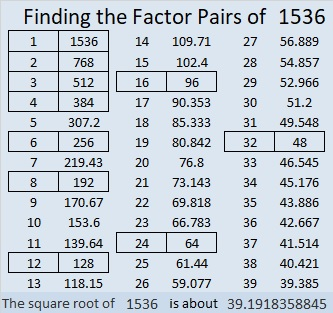# Factors of 1536 Make Sum-Difference!

### Today’s Puzzles:

1536 has 10 different factor pairs. One of those pairs adds up to 80 and a different one subtracts to give 80. Can you find the factor pairs that make sum-difference and write them in the puzzle? You can look at all of the factor pairs of 1536 in the graphic after the puzzle, but the second puzzle is really just the first puzzle in disguise. So try solving that easier puzzle first.That first puzzle is the first possible Sum-Difference puzzle. The second puzzle is only the 49th possible puzzle.

### Factors of 1536:

• 1536 is a composite number.
• Prime factorization: 1536 = 2 × 2 × 2 × 2 × 2 × 2 × 2 × 2 × 2 × 3, which can be written 1536 = 2⁹ × 3.
• 1536 has at least one exponent greater than 1 in its prime factorization so √1536 can be simplified. Taking the factor pair from the factor pair table below with the largest square number factor, we get √1536 = (√256)(√6) = 16√6
• The exponents in the prime factorization are 9 and 1. Adding one to each exponent and multiplying we get (9 + 1)(1 + 1) = 10 × 2 = 20. Therefore 1536 has exactly 20 factors.
• The factors of 1536 are outlined with their factor pair partners in the graphic below.### More About the Number 1536:

1536 is the difference of two squares in EIGHT different ways!
385² – 383² = 1536,
194² – 190² = 1536,
131² – 125² = 1536,
100² – 92² = 1536,
70² – 58² = 1536,
56² – 40² = 1536,
44² – 20² = 1536, and
40² – 8² = 1536.

1536 = 6 × 256.
256 is the 100th number whose square root can be simplified.
1536 is the 600th number whose square root can be simplified.

Here are the 501st to the 600th simplifiable square roots. If at least three square roots are consecutive, they are highlighted.